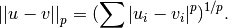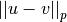# scipy.spatial.distance.minkowski¶

scipy.spatial.distance.minkowski(u, v, p)

Computes the Minkowski distance between two vectors u and v, defined asParameters : u : ndarray An n-dimensional vector. v : ndarray An n-dimensional vector. p : int The order of the norm of the difference. d : double The Minkowski distance between vectors u and v.

#### Previous topic

scipy.spatial.distance.matching

#### Next topic

scipy.spatial.distance.rogerstanimoto